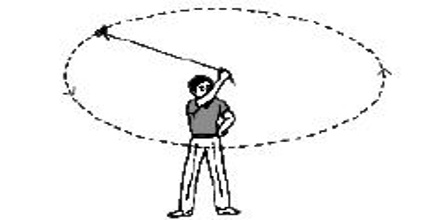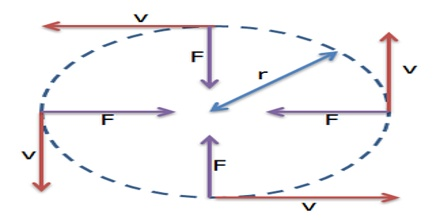# Uniform Circular Motion

Uniform Circular Motion

If you hold one end of a thread that has a stone fastened at the other end and if you rotate the thread over your head, you will see that the stone is rotating in a circular path. Now, if you just release the thread, you will never see that the stone after whirling many times has fallen on your head. Rather you will see that the stone has left your hand along the tangent at a point in the circular path as in figure (a). If you look at the figure below you will understand it clearly. When the stone is whirling over the head then at every moment, if the magnitude of the velocity is constant, then that motion will be uniform circular motion.That means, if a particle rotates around a circular path at constant speed than that motion is called uniform circular motion.

In uniform circular motion although the magnitude of velocity of the body remains unchanged, direction changes. So, velocity changes. The direction of the velocity at any point is equal to the tangent at a point in the circular path. So, a force acts in order to change the direction of velocity i.e., an acceleration acts. The direction of this acceleration is along the normal to the direction of motion and towards the centre of the circle. This acceleration is centripetal acceleration. If the acceleration would have been in different direction, then along the tangent of the circle i.e., along the direction of motion of the particle there would have a component of acceleration, consequently change of speed of the particle would occur.But when a body moves along the periphery of a circle in uniform speed then that motion of the body is uniform circular motion. Although the body in this motion rotates in uniform speed but direction at every point changes. Tangent drawn at any point along the periphery will be the direction of velocity of that point [Fig.(b)]. As the directions of tangents at different points are different, so the direction of velocity in all the time changes. That means velocity is also changing, thus there is acceleration. So, it can be said that a body travelling in circular path with uniform speed has acceleration.